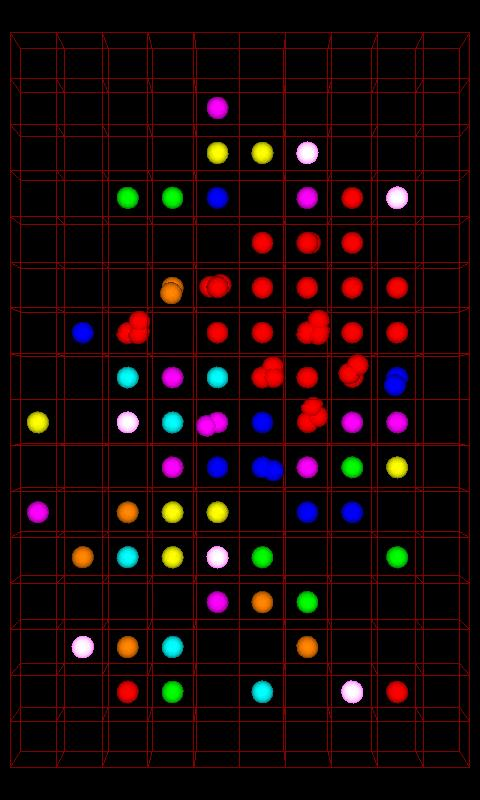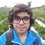# Chain Reaction - Generalized$l$ players are playing a game of Chain Reaction on a $m \times n$ grid. They start with an empty grid, and then when their turn comes they place on orb on the grid.

Here are two challenging problems:

1) What is the maximum number of moves the game can last?

2) What is the minimum number of moves the game can last?

Answer in terms of $l, m,$ and $n$.

Details and assumptions

• $l, m, n$ are integers. $l \geq 2$, and $m, n \geq 1$.
• Assume that all the players play with the objective to maximize(in case 1)/minimize (in case 2) the number of moves.
• For better clarity, you can try the above questions with the special case $l = 2, m = 6, n = 8$ here and here

I haven't solved it, so I don't know the solution. I don't even know if a (nice) solution exists, however it seems like an interesting problem to me and I hope you will like it too. Feel free to use any method(s) to get the answer - pen and paper, marker and whiteboard, programming, Wolfram|Alpha or anything else that you like!

Post below any ideas / strategies that come to your mind that could be used to maximize / minimize the number of moves.Note by Pranshu Gaba
5 years, 11 months ago

This discussion board is a place to discuss our Daily Challenges and the math and science related to those challenges. Explanations are more than just a solution — they should explain the steps and thinking strategies that you used to obtain the solution. Comments should further the discussion of math and science.

When posting on Brilliant:

• Use the emojis to react to an explanation, whether you're congratulating a job well done , or just really confused .
• Ask specific questions about the challenge or the steps in somebody's explanation. Well-posed questions can add a lot to the discussion, but posting "I don't understand!" doesn't help anyone.
• Try to contribute something new to the discussion, whether it is an extension, generalization or other idea related to the challenge.
• Stay on topic — we're all here to learn more about math and science, not to hear about your favorite get-rich-quick scheme or current world events.

MarkdownAppears as
*italics* or _italics_ italics
**bold** or __bold__ bold
- bulleted- list
• bulleted
• list
1. numbered2. list
1. numbered
2. list
Note: you must add a full line of space before and after lists for them to show up correctly
paragraph 1paragraph 2

paragraph 1

paragraph 2

[example link](https://brilliant.org)example link
> This is a quote
This is a quote
    # I indented these lines
# 4 spaces, and now they show
# up as a code block.

print "hello world"
# I indented these lines
# 4 spaces, and now they show
# up as a code block.

print "hello world"
MathAppears as
Remember to wrap math in $$ ... $$ or $ ... $ to ensure proper formatting.
2 \times 3 $2 \times 3$
2^{34} $2^{34}$
a_{i-1} $a_{i-1}$
\frac{2}{3} $\frac{2}{3}$
\sqrt{2} $\sqrt{2}$
\sum_{i=1}^3 $\sum_{i=1}^3$
\sin \theta $\sin \theta$
\boxed{123} $\boxed{123}$

## Comments

Sort by:

Top Newest

The minimum number of moves depends heavily on the starting configuration. As an example, for $l = 2, \min \{m,n\} \ge 2$, and Player 1 has only one orb on a corner, we can play the following:

1. Starting configuration has Player 1 on corner $(1,1)$.
2. Player 1 plays on $(1,1)$, splitting to $(1,2)$ and $(2,1)$.
3. Player 2 plays on $(1,1)$.
4. Player 1 plays on $(1,2)$.
5. Player 2 plays on $(1,1)$, chaining to eliminate all of Player 1's orbs.

However, the general approach is that; get cells of a player to almost reach critical mass (just to aid when they explode), and chain as many as possible together, so that they can be eliminated quickly.

The maximum number of moves is actually reached when all cells have one less than critical mass (so that they don't explode yet), and then one more move is played, triggering an infinite chain reaction.

- 5 years, 11 months ago

Log in to reply

Nice observation! To get as many moves as possible, all cells must have one less than critical mass. But then the question arises for what condition on $l, m, n$ can that state be reached? If that state can not be reached, that what is the next best option?

Note that the we are starting on an empty grid i.e. there is only one starting configuration. For $l = 2$, the minimum number of moves is less than $5$, it is in fact $3$.

1. Player 1 plays on $(1, 1)$.
2. Player 2 plays on either $(1, 2)$ or $(2, 1)$.
3. Player 1 plays on $(1, 1)$, eliminating Player 2

- 5 years, 11 months ago

Log in to reply

I think maximum no of moves will be independent of no of players since we have to make all orbs reach Critical mass so I'm getting the answer: 3mn-2m-2n not calculated min moves till now.

PS: This will be true when no of players is a factor of total squares, otherwise it's very difficult

- 5 years, 11 months ago

Log in to reply

Yes, you are correct; in order to have maximum moves, we must try to make all orbs reach critical mass. Can you prove that if no. of players $l$ is a factor of total number of squares $m \times n$, then it is always possible to make all orbs reach critical mass? Also is it true that if $l$ is not a factor of $m \times n$, then it is always impossible to make all orbs reach critical mass?

- 5 years, 11 months ago

Log in to reply

×

Problem Loading...

Note Loading...

Set Loading...